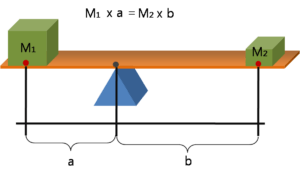iSoul In the beginning is reality.

# Measuring mass and elaphrance

A mass may be measured by a scale with a fulcrum, such as this:If the mass of M1 is a standard mass such as a kilogram, then the gravitational mass of M2 can be determined from the law of the lever as follows:

M2 = M1 × a ÷ b.

Such a scale can also be used to measure the inverse mass, 1/M2, which I’m calling the elaphrance, L2:

L2 = 1/M2 = b ÷ (M1 × a) = L1 × b ÷ a.

The mass and the elaphrance are related properties of a physical body. They can be measured together. The scale for elaphrance is the inverse of the scale for mass: the scale for minimum to maximum mass corresponds to the scale for maximum to minimum elaphrance.